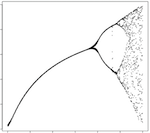# Prime Number Digit Frequencies

Today I became curious about the relative frequencies of the digits that appear in prime numbers (in base 10).

Naturally, I wrote a little program to show the answer - digit-freq2:

Continue reading Prime Number Digit Frequencies...

# How Many Primes Are In A Slice Of Pi?

Questions:

• What is the number of primes per n-slice of the decimal part of pi?
• What does the graph look like?
• Are there many overlapping primes (i.e. start at the same position in the slice)?

Continue reading How Many Primes Are In A Slice Of Pi?...

# Alternatives to the Logistic EquationYesterday, I decided to plot the bifurcation diagram of the logistic equation.  This is a famous plot from the 70s, with which many geeks will be familiar.  It shows that simple systems can switch into "chaos mode" and begin to bifurcate wildly.

tl;dr: bifurcation.R

Continue reading Alternatives to the Logistic Equation...